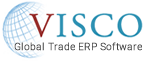(845) 383-3800SEE THE SOFTWAREREQUEST A DEMOCONTAINER TRACKING

# How to Calculate Landed Cost## How to Calculate Landed Cost

Landed Costs are used to define the true actual cost to bring goods into inventory.  This is generally considered a more meaningful value when calculating profitability on sales than using just the FOB cost or the cost paid the supplier.

Calculating Landed costs is of extreme importance to a company importing and distributing goods because understanding the additional costs that go into the product beyond the FOB cost can often make the difference between selling at a loss or at a profit.

Calculating Landed Cost Using An Example:

The best way to understand the calculation of landed costs is to use an example.  In this case, we will use the example of a company buying cases of black trash cans from China and selling them in the United States.

Material Cost:

The foundation of the landed cost is almost always going to be the FOB cost or the cost of the product itself.  The calculation for Material cost is the price per unit as billed by the supplier.  In this case, lets say that the trash cans cost \$3.00 each.

Duty:

The cost of duty is normally the second or third most expensive component of the landed cost.  This is calculated by using the value of the goods being imported into the country multiplied by the duty rate.  In this case, let’s say that the duty rate is 5% so for the trash cans it would be:

\$3.00*.05=\$0.15 additional cost per trash can

Ocean Freight:

Since most goods are imported via Ocean Freight we will use this example when including freight cost in the landed cost calculation.  Note that the way the freight cost will be calculated will vary depending on the type of product being imported and the way that product is valued.  If a product is being bought and sold based on weight or if the container is typically filled to max capacity by weight before it is filled to max capacity by volume then you would want to distribute the Ocean Freight cost based on weight.

However, if the goods are typically priced per piece or per case and the container is filled to capacity by volume before it is filled to capacity by weight then you would want to distribute the Ocean Freight Cost by volume instead.  In our example of trash cans, they will fill the container by volume before they will fill it by weight so we will use the by volume calculation.

To be able to distribute the cost of ocean freight by volume we first need to know the CBM calculation on each of the goods in the container.  In the case of the trash cans, they are packaged 12 PCS to a case and we have the case dimensions of Length of 96 cm, Width of 42 cm, Height of 24 cm=96,768 cubic cm or 0.096768 CBM per case.

In our example, lets say that the container is filled with 500 cases of black trash cans and 200 cases of white trash cans and both colors have the same carton dimensions.  The cost of the Ocean Freight is \$3000.  Since the black trash cans are taking up more of the container, we want to make sure that they also get more of the cost.  The calculation here would be:

Black=500*0.096768=48.384 CBM taken up by the black trash cans

White=200*0.96768=19.3536 CBM taken up by the white trash cans

Total CBM=67.7376

Total Ocean Freight Cost=\$3000

Total cost per CBM: \$3000/67.7376=\$44.289

Total Ocean Freight Cost of black trash cans: \$44.289 cost per CBM*48.384 CBM taken up by black trash cans=\$2,142.88

Total Ocean Freight Cost per case of the black trash can: \$2,142.88/500=\$4.29

Ocean freight cost per trash can: \$4.29/12 (12 PCS per case)=\$0.36

Drayage/Inland Freight:

If trucks are being used to transport the goods from the port to the warehouse then this cost should also be considered in the landed cost calculation.  The Drayage component of the landed cost calculation is typically handled in the same way that the Ocean Freight calculation is handled.  In our example, lets say that it cost \$800 in trucking cost to bring the container from the port to the warehouse and we want to distribute that cost by CBM as well:

Black=500*0.096768=48.384 CBM taken up by the black trash cans

White=200*0.96768=19.3536 CBM taken up by the white trash cans

Total CBM=67.7376

Total Drayage Cost=\$800

Total cost per CBM: \$800/67.7376=\$11.81

Total Drayage Cost of black trash cans: \$11.81 cost per CBM*48.384 CBM taken up by black trash cans=\$571.42

Total Drayage Cost per case of black trash can: \$571.42/500=\$1.14

Drayage cost per trash can: \$1.14/12 (12 PCS per case)=\$0.10

Total Landed Cost:

Material: \$3.00

Duty: \$0.15

Ocean Freight: \$0.36

Drayage: \$0.10

Landed Cost per Trash Can=\$3.88

BLOG CATEGORIES
Related Articles